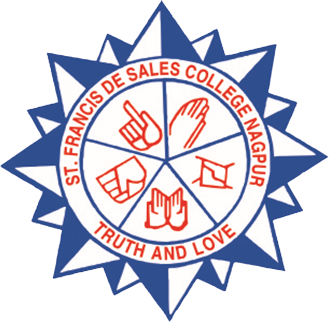# ST. FRANCIS DE SALES COLLEGE NAGPUR, India

## Courses

UG Level

Bachelor of Science( B.Sc.) degree course with Physics as one of the Subject.

Following combinations are available

1.) Bachelor of Science( B.Sc.) in Physics, Mathematics , Chemistry (PMC)

2.) Bachelor of Science( B.Sc.) in Physics, Mathematics , Electronics (PME)

3.) Bachelor of Science( B.Sc.) in Physics, Mathematics , Computer Science (PMC/S)

Total Intake capacity = 120

B.Sc. Course Pattern : Semester pattern Six Semester (3 year)

Each Semester has

02 Theory Paper each of 50 Marks

01 Practical of 30 Marks

One Internal Assignment of 10 marks for each theory paper

Courses at PG Level

Masters of Science (M.Sc.) in Physics (Non-Grant)

Intake capacity = 22

Specialization /Elective paper : Materials Science

Subject Centric Core Course Papers:

Sem-III Nanoscience and Nanotechnology

Sem-IV Experimental Techniques in Physics

Ph.D. in Physics

M.Sc. Physics (CBCS)

(Semester pattern 2 years program)

Programme Outcomes, Programme Specific Outcomes and Course Outcomes

Program outcomes:

PO-1: To solve the multifaceted scientific problems and become knowledgeable experts at global level.

PO-2: To apply knowledge of physics to solve advanced scientific problems

PO-3: To conduct scientific experiment techniques and interpretation of the scientific data.

PO-4: To improve and implement new skills for future in teaching and research

PO-5: To compete national and international level competition.

PO-6: To apply appropriate knowledge to help society.

Program specific outcomes:

After completion of Post- graduation students will have

– Elementary and advanced knowledge of Mathematical Physics, Electronics, Electrodynamics, Statistical Physics, Classical physics, Quantum physics, Solid state physics, Material science and Nano material physics.

– Knowledge of designing and operate scientific experiments and interpret the experimental data.

– Basic knowledge of research and development.

Course Outcomes:

Semester I

Mathematical Physics-

This paper enables the students:

– To understand Fourier Series, Laplace transformation , Tensor

– To Differential equation, matrix, vectors and special functions like Hermite, etc.

Complex Analysis and Numerical Methods-

– To be able to understand Complex Numbers and Singularities

– To be able to understand Numerical method , Bisection method, false position method, iteration method, Newton-Raphson method etc

– To be able to understand Lagrange’s interpolation and Runge Kutta methods for solving ordinary differential equations

Electronics-

– To be able to understand Electronics Semiconductor discrete devices, Opto-electronic devices

– To be able to understand Transistor as amplifier , gates ,Convertors and Communication Electronics

Electrodynamics I-

– To understand the basic laws of Electrostatics

– To get knowledge about Magnetostatics and time varying field

Seminar – I

– To provide a platform for enhancing their self-confidence and improving communication skills, learning new topics for knowledge, also to convey what their ideas are about the topics and to encourage discussion among students.

Practical 1&2

To gain concepts and hands on basic programming related ideas to be able to solve about computational problems. Also to learn about basic electronics circuit practical’s to apply in real life situations.

Semester II

Quantum Mechanics I

– To understand Schrodinger equation, quantum mechanical operators

– To be able to understand Hermitian operators, Dirac’s bra-ket notations

– To be able to understand Solution of Schrodinger equation for simple problems, Angular momentum operator and spin angular momentum

Classical Mechanics-

– To be able to understand Basics of Elementary principles of mechanics of a particle

– To understand Hamiltonian formalism, Central force motion and Rigid body dynamics

Statistical Physics-

– To understand the fundamentals of classical statistical mechanics, Bose-Einstein statistics , Fermi dirac statistics, Maxwell Boltzmann statistics

– To be able to understand Phase transition , ordered disordered transition , Ising model etc

Electrodynamics II

– To get knowledge about Scalar and vector waves, Symmetries of Maxwell equations

– To be able to understand Motion of a charge in EM fields, Wave guides( TE,TM and TEM )

Seminar – II

– To provide a platform for enhancing their self-confidence and improving communication skills, learning new topics for knowledge, also to convey what their ideas are about the topics and to encourage discussion among students.

Practical 3&4

To gain advanced concepts in programming (PYTHON/C/C++) related ideas to be able to solve about computational problems.

Also to learn about advanced Physics concepts practically for better understanding of topics.

Semester III

Quantum Mechanics II

– To understand the basic concept of Time independent and time dependent perturbation theory, Zeeman effect, Stark effect, different and approximation methods to solve physics problem

– To understand quantum theory behind many particle system , scattering phenomena , Dirac theory

Solid state physics and spectroscopy

– To be able to understand crystal structure , their symmetry and defects in solid

– To be able to understand dielectric, piezoelectric , ferroelectric and pyroelectric properties of materials

– To be able to understand Atomic and Molecular spectra and their application

Material Science -I

– To develop understanding about equilibrium, Phase diagram of various reaction and its application , crystal growth kinetics

– To be able to understand basics about diffusion in solids and solid state ionics

– To get detailed knowledge of solid state device like Lithium ion battery , solar cell and fuel cell